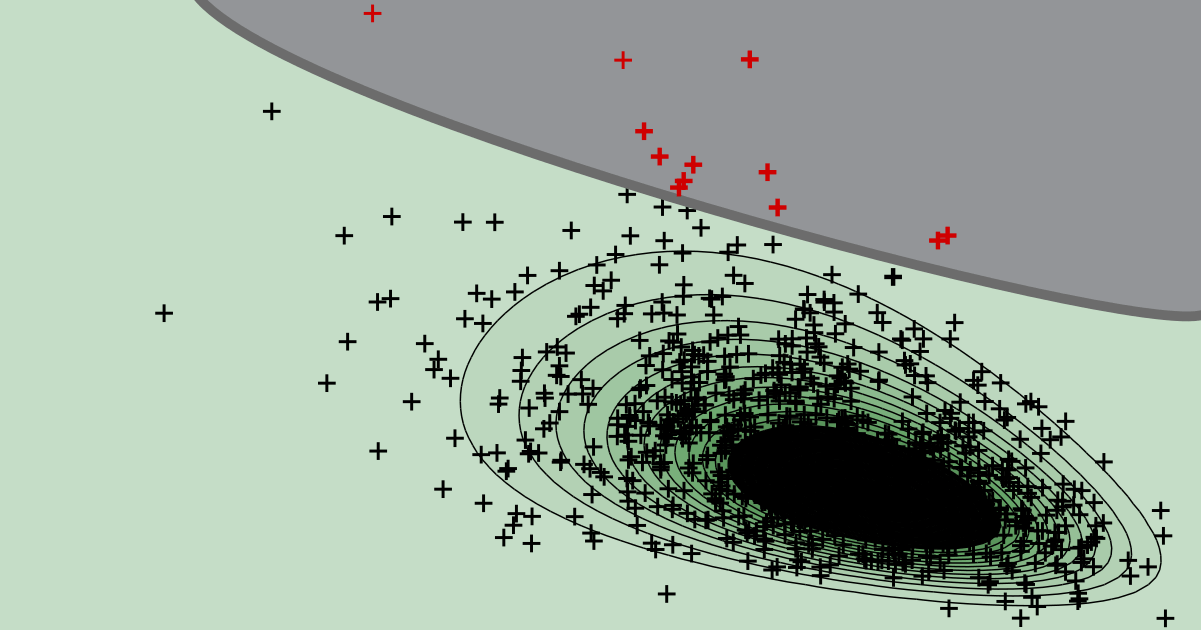# Reliability analysis

Structural reliability analysis aims at evaluating the probability of failure of a structure or system. To evaluate the probability of failure an integral over the sample space must be computed.

Reliability analysis

## What is FORM?

FORM is a structural reliability analysis method that approximates the probability of failure by linearizing the limit state function around the design point.

Reliability analysis

## What is the most likely failure point?

It is the point in the failure domain that has the smallest distance to the origin in standard Normal space. This point is also referred to as design point.

Reliability analysis

## How is the reliability index linked to the probability of failure?

The reliability index is directly linked to the probability of failure through the negative of the inverse CDF of the standard Normal distribution.

Reliability analysis

## What is the 'safety margin' in structural reliability analysis?

The difference between the capacity and the demand is the safety margin.

Reliability analysis

## What is the 'basic structural reliability problem'?

When the limit state function can be expressed as the difference between capacity and demand, the problem is sometimes referred to as basic reliability problem.

ONLINE TOOLReliability analysis

## Post-processing of Monte Carlo simulation

Use our web app to quantify the uncertainty about the probability of failure based on a conducted Monte Carlo simulation.
try it ...

Reliability analysis

## Why should you avoid Normal confidence intervals for Monte Carlo simulation?

The underlying distribution can be highly skewed, even if the total number of samples in the Monte Carlo simultion is very large. This is why the Normal approximation often performs poorly in practice.

Reliability analysis

## Why to avoid the coefficient of variation in the context of a Monte Carlo simulation?

The distribution quantifying the uncertainty about the probability of failure can be highly skewed, even for a large number of samples. The coefficient of variation is easier to interprete for symmetric distributions.

Reliability analysis

## How to interpret the outcome of a Monte Carlo simulation if no failures occurred?

Even if Monte Carlo simulation returns not a single sample in the failure domain, we can still quantify the uncertainty about whether a specified target reliability level is maintained.

Reliability analysis

## How to assess the uncertainty in the outcome of a Monte Carlo simulation?

We explain a Bayesian post-processing step for MCS. It is ideally suited to quantify the uncertainty and to evaluate credible intervals for the probability of failure.

Reliability analysis

## How large is the statistical uncertainty in the estimate of a Monte Carlo simulation?

For a given probability of failure, the variance and coefficient of variation of the Monte Carlo estimate can be evaluated analytically. From this, the total number of samples required to maintain a target coefficient of variation can be deduced.